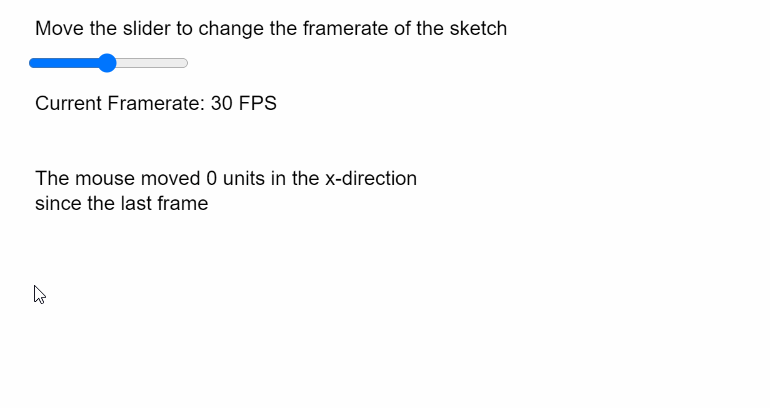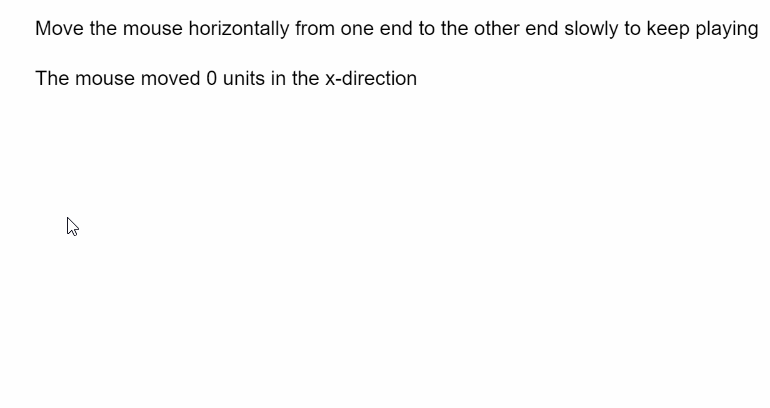# p5.js | Mouse movedX

• Last Updated : 31 May, 2020

The movedX variable in p5.js contains the horizontal movement of the mouse since the last frame of the sketch. A positive value indicates that the mouse moved to the right and a negative value indicates that it moved to the left in the last frame.

Syntax:

```movedX
```

The program below illustrates the movedX variable in p5.js:
Example 1:

## javascript

 `function` `setup() { ` `  ``createCanvas(400, 300); ` `  ``textSize(16); ` ` `  `  ``fpsSlider = createSlider(1, 60, 30, 1); ` `  ``fpsSlider.position(20, 40); ` `} ` ` `  `function` `draw() { ` `  ``clear(); ` `  ``text(``"Move the slider to change the framerate "``+ ` `       ``"of the sketch"``, 20, 20); ` ` `  `  ``// Set the framerate according to the slider ` `  ``frameRate(fpsSlider.value()); ` `  ``text(``"Current Framerate: "` `+ fpsSlider.value() + ``" FPS"``, 20, 80); ` ` `  `  ``// Use the movedX property ` `  ``text(``"The mouse moved "` `+ movedX + ``" units in the x-direction"``, 20, 140); ` `  ``text(``"since the last frame"``, 20, 160); ` `} `

Output:Example 2:

## javascript

 `let gameOver = ``false``; ` ` `  `function` `setup() { ` `  ``createCanvas(600, 300); ` `  ``textSize(16); ` `} ` ` `  `function` `draw() { ` `  ``clear(); ` `  ``text( ` `    ``"Move the mouse horizontally from one end to "``+ ` `    ``"the other end slowly to keep playing"``, ` `    ``20, ` `    ``20 ` `  ``); ` `  ``if` `(!gameOver) { ` ` `  `    ``// Use the movedX property to display the ` `    ``// amount of mouse moved ` `    ``text(``"The mouse moved "` `+ movedX + ``" units in "``+ ` `         ``"the x-direction"``, 20, 60); ` ` `  `    ``// Get the absolute amount of mouse moved ` `    ``// and finish the game is it goes too fast ` `    ``if` `(abs(movedX) > 3) gameOver = ``true``; ` `  ``} ``else` `text(``"You moved too fast! Refresh to try again"``, 20, 80); ` `} `

Output:My Personal Notes arrow_drop_up
Recommended Articles
Page :